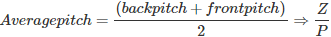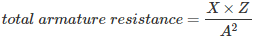# MCQs on Electrical Machines

##### Page 3 of 114. Go to page 1 2 3 4 5 6 7 8 9 10 11 12 13 14 15 16 17 18 19 20 21 22 23 24 25 26 27 28 29 30 31 32 33 34 35 36 37 38 39 40 41 42 43 44 45 46 47 48 49 50 51 52 53 54 55 56 57 58 59 60 61 62 63 64 65 66 67 68 69 70 71 72 73 74 75 76 77 78 79 80 81 82 83 84 85 86 87 88 89 90 91 92 93 94 95 96 97 98 99 100 101 102 103 104 105 106 107 108 109 110 111 112 113 114
01․ DC machine windings are
full pitched
short pitched
either of these
none of the above

DC machine windings are full pitched winding only because it offers maximum induced emf within the coil. No requirement of short pitching of coils like alternator.

02․ Which of the following represents the commutator pitch?
Number of conductors spanned by one coil at the back end of the armature.
Number of conductors spanned by one coil at the front end of the armature.
The distance between the staring of first coil and its next successive coil
Number of commutator segments between two successive coils.

The definitions of different types of pitches are
1. Back pitch: Number of conductors spanned by one coil at the back end of the armature.
2. Front pitch: Number of conductors spanned by one coil at the front end of the armature.
3. Resultant pitch: The distance between the staring of first coil and its next successive coil.
4. Commutator pitch: Number of commutator segments between two successive coils.
For lap winding YC is the difference of YB and YF where as for wave winding it is the sum of the two.

03․ In a dc machine 4 pole lap winding is used. The number of parallel paths are?
2
4
1
8

In lap winding number of parallel paths = number of poles = 4. For Wave winding it will be equal to 2.

04․ In a dc machine 6 pole wave winding is used. The number of parallel paths are?
6
4
2
1

In wave winding / simplex wave winding number of parallel paths = always 2 (irrespective of number of poles).

05․ Inter pole winding is connected in-------------------------- ?
series with armature
series with main poles
parallel with armature
parallel with main poles

Interpolars are small narrow commutating poles connected in series with armature. It produce an extra flux to neutralize the reactance m.m.f. produced at the time of commutation process.

06․ In a lap winding dc machine number of conductors are 100 and number of parallel paths are 10. Find the average pitch
10
100
50
1(∵ front pitch = Yb+1, back pitch = Yb -1 )

07․ Lap winding is prefered for which type of machines?
low current and low voltage
high current and high voltage
high current and low voltage
low current and high voltage

Number of parallel paths are more in case of lap winding than wave winding. So summation of currents at the output is more. Therefore it is preferred for high current and low voltage rated machines.

08․ Wave winding is prefered for which of the following rating?
low current and low voltage
high current and high voltage
high current and low voltage
low current and high voltage

Number of parallel paths in wave winding is only 2. Therefore is used for low current and high voltage rated machines. Summation of currents in parallel paths is less than wave winding.

09․ Equalizer rings are used in which of the followings?
lap winding
wave winding
both 1 and 2
none of the above

Equalizer rings are essential in lap winding than wave winding because of more no of parallel paths in lap winding. As in lap winding no of parallel path is more, there would be severe sparking at brushes due to difference in currents in different parallel paths. But in wave winding no of parallel paths is two and the sparking at commutation is less. So, equalizer rings are used in lap winding to avoid any unequal distribution.

10․ In a 2 pole lap winding dc machine , the resistance of one conductor is 2Ω and total number of conductors is 100. Find the total resistance
200Ω
100Ω
50Ω
10Ω

Total resistance depends upon no of parallel path. In lap winding parallel path is no of poles and here it is two. Half of conductor are in series i.e. 50 in series and rest of 50 in series and they are parallel together. 50 no 2Ω in series = 100Ω. When two such paths are parallel their equivalent will be 50 Ω. Numerically it can also be stated,X = Resistance of one conductor Z = Total number of conductors A = No of parallel paths

<<<12345>>>